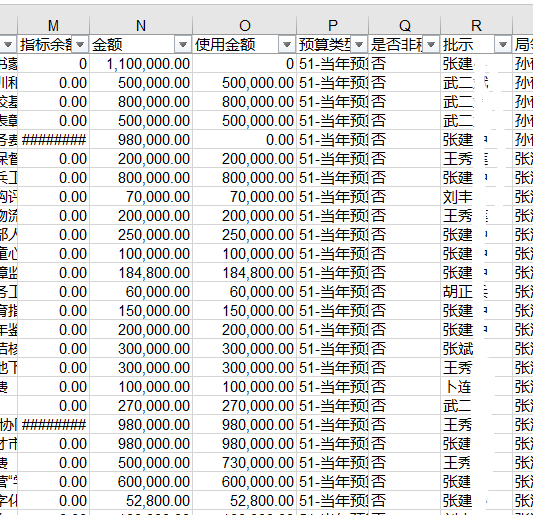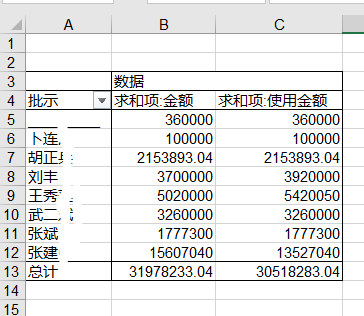2019-12-17 13:42

# 【python3.8字典问题请教】对Excel中数据提取，相同项对应值相加，写入新sheet表``````print('Opening workbook...')
sheet = wb.get_sheet_by_name('Sheet1') #获取工作表
nameData = { }  #创建字典
for row in range(2,sheet.max_row+1):  #从第二行循环到最后一行
JE = sheet['N'+str(row)].value
name = sheet['E'+ str(row)].value
SY = sheet['O'+str(row)].value  #存储每一列的数据
#字典结构nameData['姓名'][金额][使用金额]
nameData={name,(JE,SY)}
``````

【问题】

• 写回答
• 好问题 提建议
• 关注问题
• 收藏
• 邀请回答

#### 4条回答默认 最新

•吃鸡王者 2019-12-17 14:15
已采纳

建议用pandas来处理
具体如下：
如果没有安装pandas要先安装pandas模块（pip install pandas）

``````import pandas as pd
data=data[['批示','金额','使用金额']]
data=data.groupby(by='批示',as_index=False).sum()
data.columns=['批示','求和项：金额','求和项：使用金额']               #data 就是你要的数据表

``````

或者

``````import pandas as pd
data=data[['批示','金额','使用金额']]
data=data.groupby(by='批示').sum()
data.columns=['求和项：金额','求和项：使用金额']               #data 就是你要的数据表

``````
已采纳该答案
评论
解决 无用
打赏 举报
•幸福丶如此 2019-12-17 14:01

这个使用pandas处理起来比较简单，这个是我根据excel简单些的一个代码，不知道怎么样，可以先试试

``````import pandas as pd
nameData = { }  # {'批示人名':{"amount":[],'usageamount':[]}}

for name,amount,usageamount in zip(df['批示人'],df['金额'],df['使用金额']):
if name not in nameData.keys():
nameData[name] = {"amount":[float(amount)],"usageamount":[float(usageamount)]}
else:
nameData[name]["amount"].append(float(amount))
nameData[name]["usageamount"].append(float(usageamount))

print(nameData)
for k,v in nameData.items():
print("批示人：%s , 金额：%s，使用金额：%s" %(k,sum(v['amount']),sum(v['usageamount'])))
``````
评论
解决 无用
打赏 举报
•海鸥软件 2019-12-17 14:15

加载xlwings 用它操作excel很方便，没有就在线pip install xlwings安装，使用方法网上有很多，感觉目前它操作excel表是最方便的一种，可以读写块，自己试试

评论
解决 无用
打赏 举报
•放风喽 2019-12-17 16:34

换个思路，使用VLOOKUP
找到对应的数值，相加

评论
解决 无用
打赏 举报